# Lets excel in Excel - Funda # 2

CA Nayan Gupta , 16 May 2016

Hey friends!!

Welcome back. It was great to receive a prodigious response on my earlier post on “Excel Fundas”. I’m glad that people found it useful.

For the people, who missed out my earlier post, this series of posts is an attempt to share the useful tips on the most used software in financial analysis viz. MS excel. The focus of entire exercise is to learn the best approach of performing certain tasks in excel, in a way to reduce time taken and improve accuracy.

Let us learn another simple yet useful function in excel.

SUM V. SUBTOTAL

Oh, are they really different? Indeed they are. SUM and SUBTOTAL are two ways of adding numbers. Most of us generally use the SUM function for adding the values and are not really aware the utility of the SUBTOTAL function. If you are not aware about how to apply these two functions, please google it or take help of excel F1 help. I am not going into that. The focus here is to understand when SUBTOTAL function should be preferred.

Let us try to understand this the following set of data.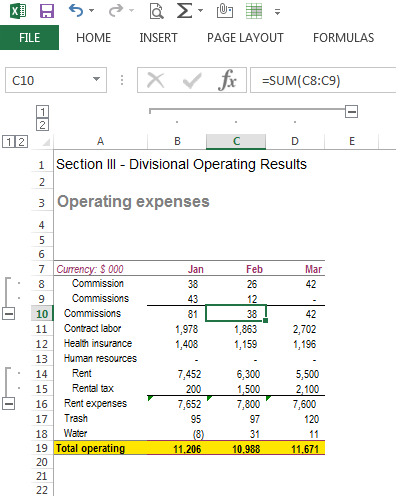As we can see, there are various subgroupings done in the data. When we see rent expenses in row # 16, it is sum total of rows # 14 and 15. Similar is the case with row # 10. I have taken very small data in this example. There might be hundreds of such grouping in a single set of data.

To calculate ‘total operating expenses’ in row # 19, first of all rows # 8 and 9 should be summed in row # 10 and then this sum is to be included in the sum for ‘total operating expense’, right?

Let us consider using both SUM and SUBTOTAL functions, to appreciate the difference between the two.

Scenario 1- SUM Function

Refer screenshot below: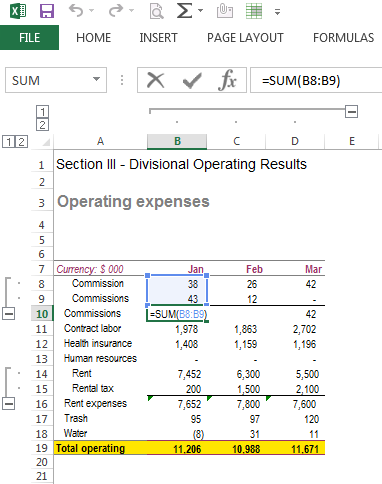As we can observe, sum formula is used in this case to sum rows # 8 and 9. So when we calculate “total operating expenses in row # 19, the sum formula would look something like this (refer screenshot below):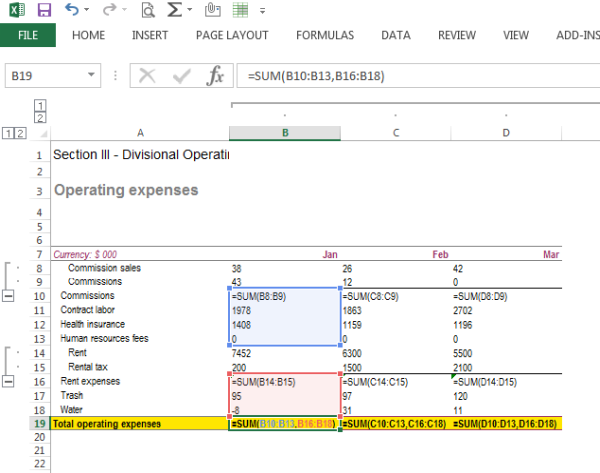As we can note, the sub-grouped items i.e. rows # 8, 9, 15 and 16 are excluded from the SUM formula so as to avoid double counting of data. Had the entire range i.e. B8:B18 been included in the SUM formula, the value for rows # 8, 9, 15 and 16 would have been counted twice.

Now, let us try to do it our way ;)

Scenario 2: Using SUBTOTAL function

Let us see the data again:Let us apply SUBTOTAL functions in rows # 10 and 16. Refer screenshot below: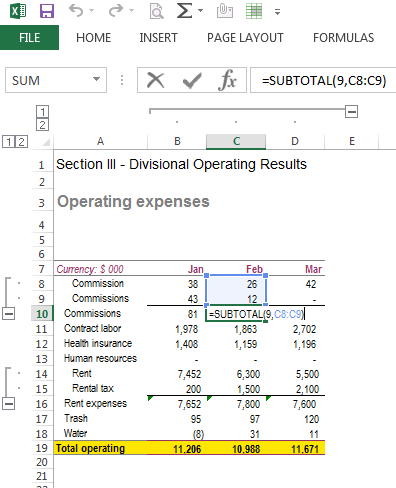Now let us see how using the SUBTOTAL function in row # 19 (total operating expenses) makes our task much easier and methodological. Refer screenshot below: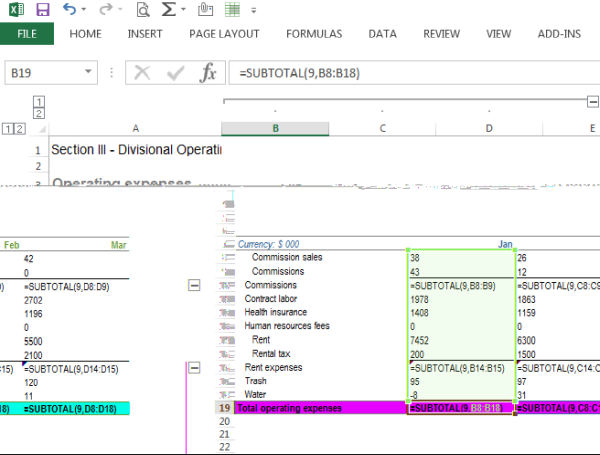So, when we apply the SUBTOTAL formula in row # 19 (total operating expenses), we simply selected the entire range B8:B18 and as we can see (refer screenshot below), both the methods give the same results.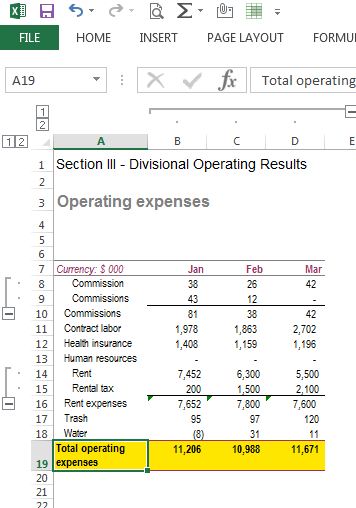So, how does SUBTOTAL function does? It ignores all the cells in the range containing SUBTOTAL function, while adding the range. So, when we applied SUBTOTAL function in cell B19, the cells B10 and B16, containing the SUBTOTAL formulas are automatically excluded from the ultimate sum. Now, we don’t have to manually select the cells to be summed.

Now, consider this for data running into ‘000s of lines to appreciate its application in financial analysis. This is a very practical case as Financial Due Diligence/ Financial Analysis/ Financial modelling is all about making the maximum sense out of the given bulk data.

So, here goes, why to use SUBTOTAL function?

1. Avoid errors of duplicate counting
2. Saving time
3. Easy modification in analysed data, i.e. adding/removing subtotals without making any change in ultimate formula.

CAUTION: Be cautious while using the SUBTOTAL formula, it excludes the cells containing SUBTOTALs only, not any other functions including SUM. So, if we are using SUBTOTAL formula, we have to consistently use SUBTOTAL function only. We cannot use blend of SUM and SUBTOTAL functions. Refer example below where we replaced SUBTOTAL formula in row # 10 with SUM formula. As we can see, the total operating expenses as appearing in row # 19 changed and row # 10 is not excluded while calculating the sum in row # 19.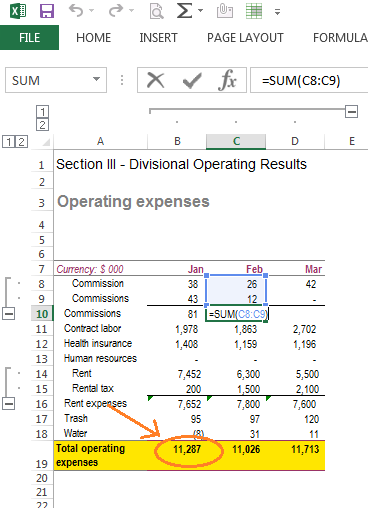Hope you find this useful. Practice on some demo data to get better feel of it.

Cheers!

CA Nayan Gupta
(Finance Manager, Overcart.com)
Category Others   Report

12 Likes   48 Shares   14706 Views

### Popular ArticlesCCI Articles